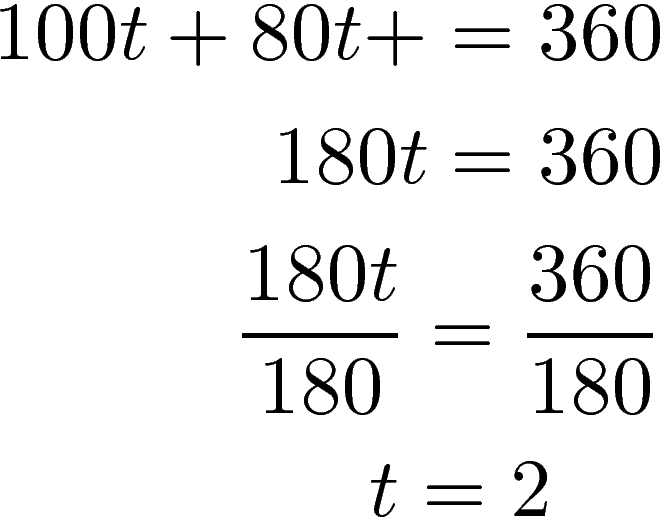# Uniform Motion Equations

A uniform motion equation involves trying to make calculations when an object(s)is moving at a constant rate. The formula for this type of equation is below.

rate * time = distance

Generally, you want to make a table that includes all of the known information. This allows you to determine what the unknown information is that needs to be solved. Below is a table that you can use.

Rate            * Time            = Distance

Let’s go through some examples

Example 1

Dan and William are riding bicycles. Dan’s speed is 4 kph faster than William’s speed. It takes William 1.5 hours to reach the beach while it takes Dan 1 hour. Find the speed of both bicyclists.

Here is what we know

• Dan is 4 kph faster than William
• It takes Dan 1 hour to get to the beach
• William is 4 kph slower than Dan
• It takes William 1.5 hours to get to the beach

We will now setup our table

Rate            * Time            = Distance
Dan  r + 4  1  1(r + 4)
William  r  1.5  1.5r

We will now solve this equation by placing Dan’s information on one side of the equation and William’s information on the other side of the equation. Below is the solutionWe now know what r is so we need to plug this into the table to get the answers

Rate            * Time            = Distance
Dan  8 + 4 = 12  1  1(8 + 4) = 12
William  8  1.5  1.5(8) = 12

The speed of Dan was 12kph while the speed of William was 8kph. This first example was two people traveling the same distance. The next example will be two people travel a different distance.

Example 2

Jenny is traveling to meet her brother. She travels from Saraburi to Chang Mai while her brother travels from Chang Mai to Saraburi. They meet in Bangkok. The distance from Saraburi to Chang Mai is 620km. It takes Jenny 2 hours to get to Bangkok while it takes the brother 7.5 hours to get there. Jenny’s brother’s average speed is 30kph faster than hers. Find the average speed for both people.

The table below captures all of our information

Rate            * Time            = Distance
Jenny r  2  2r
Brother  r + 30  7.5  7.5(r + 30)
620

To solve this problem we combine the information about Jenny and her brother and set this information to equal 620 which is the total distance. Below is the solved equation.We can now place this information in our table.

Rate            * Time            = Distance
Jenny 41.57  2  2(41.57) = 83.14
Brother  41.57 + 30 = 71.57  7.5  7.5(41.57 + 30) = 536.78
620

Jenny average speed was 41.57kph while her brother’s speed was 71.57kph. If you add up the distance traveled it will sum to 620.

Our final example will look at determining the time travel when we know the rate of the two objects.

Example 3

A husband and wife both leave their home. The wife travels east and the husband travels west. Wife travels 80kph while the husband travels 100kph. How long will they travel before they are 360km apart?

Below is what we know

Rate            * Time            = Distance
Husband 100  t 100t
Wife 80 t  80t
360

To solve this we combine the wife and husband information on one side of the equation and put the total distance traveled on the other side. The solution is below.We place our answer inside our table

Rate            * Time            = Distance
Husband 100  2 100(2) = 200
Wife 80 2  80(2) = 160
360

It takes two hours for the wife and husband to be 360km apart.

Conclusion

Understanding uniform equations involve determining first what you know and then determining what the problem wants you to figure out. If you follow this simple process and are able to identify when an equation involves a uniform application it should not be difficult to find the solution.

## 1 thought on “Uniform Motion Equations”

1.Deshna jain

Please tell me what is the equation of uniform motion